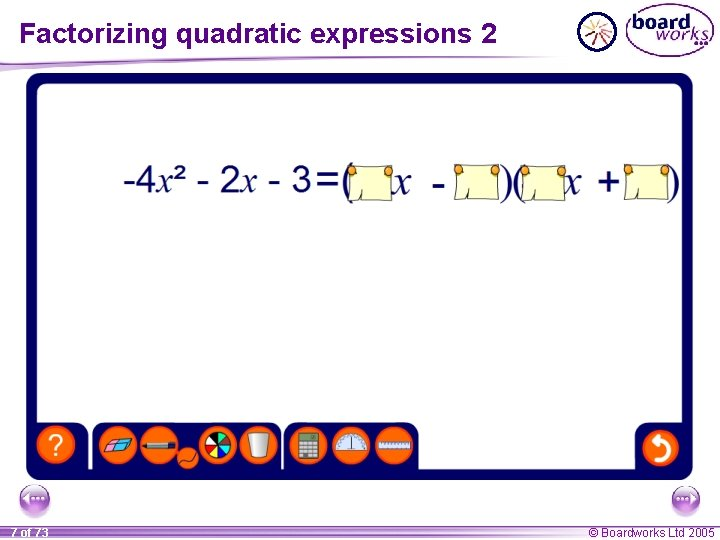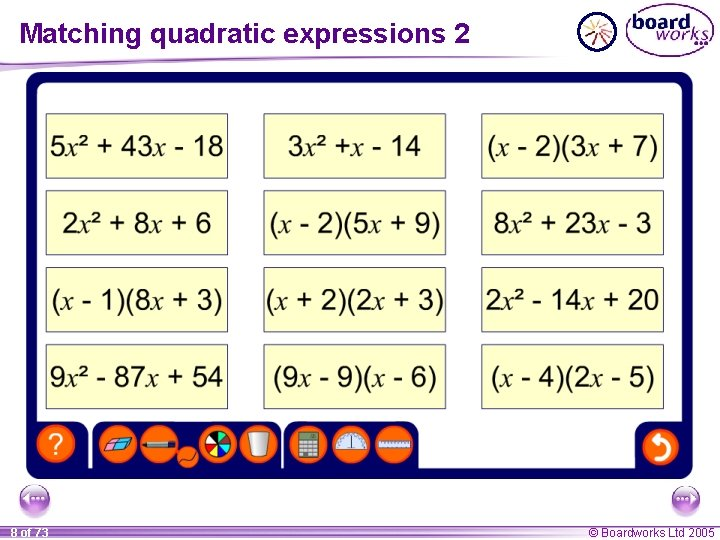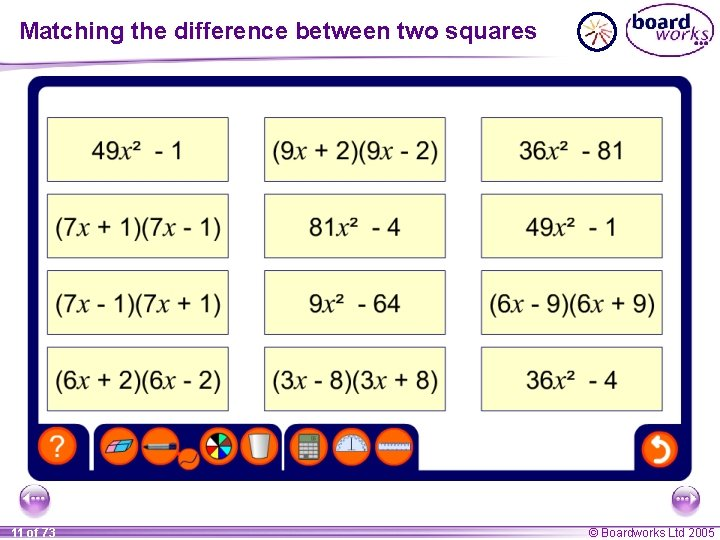• Slides: 11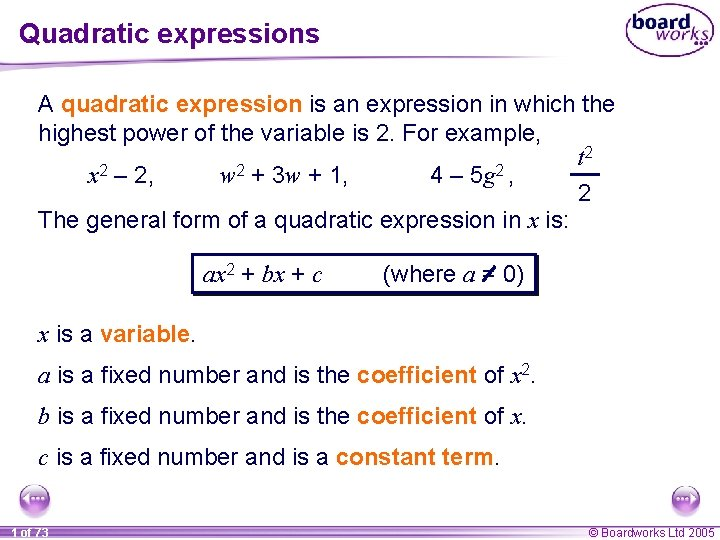Quadratic expressions A quadratic expression is an expression in which the highest power of the variable is 2. For example, 2 t x 2 – 2, w 2 + 3 w + 1, 4 – 5 g 2 , 2 The general form of a quadratic expression in x is: ax 2 + bx + c (where a = 0) x is a variable. a is a fixed number and is the coefficient of x 2. b is a fixed number and is the coefficient of x. c is a fixed number and is a constant term. 1 of 73 © Boardworks Ltd 2005Factorizing expressions Remember: factorizing an expression is the opposite of expanding it. Expanding or multiplying out a 2 + 3 a + 2 (a + 1)(a + 2) Factorizing Often: When we expand an expression we remove the brackets. When we factorize an expression we write it with brackets. 2 of 73 © Boardworks Ltd 2005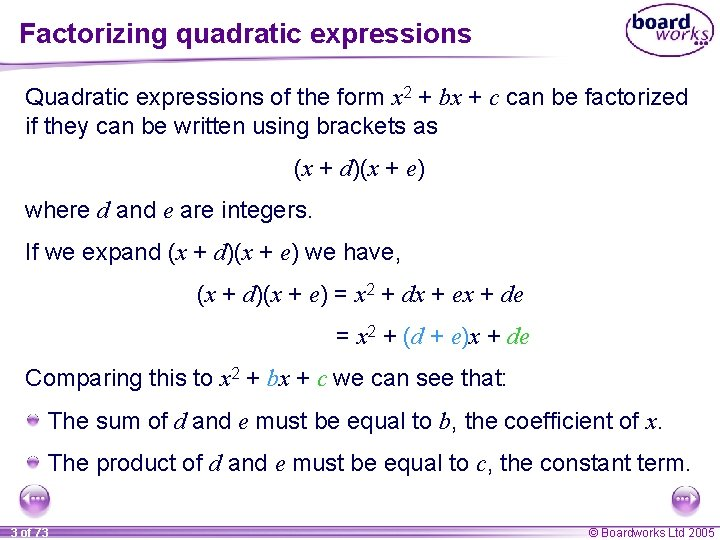Factorizing quadratic expressions Quadratic expressions of the form x 2 + bx + c can be factorized if they can be written using brackets as (x + d)(x + e) where d and e are integers. If we expand (x + d)(x + e) we have, (x + d)(x + e) = x 2 + dx + ex + de = x 2 + (d + e)x + de Comparing this to x 2 + bx + c we can see that: The sum of d and e must be equal to b, the coefficient of x. The product of d and e must be equal to c, the constant term. 3 of 73 © Boardworks Ltd 2005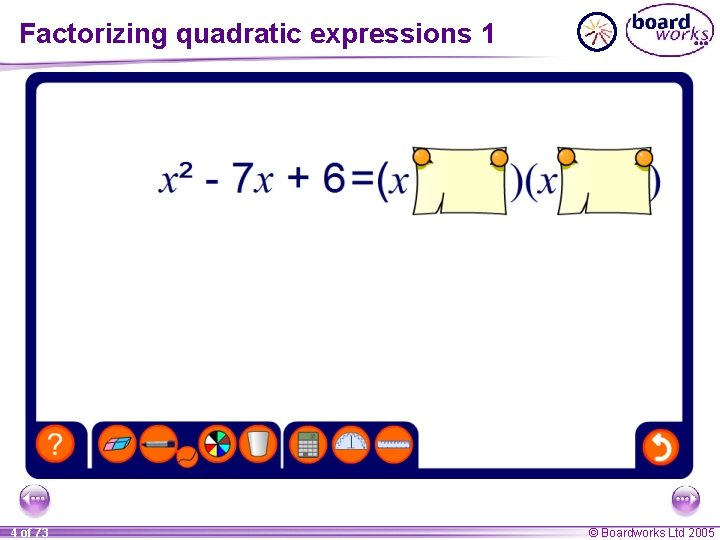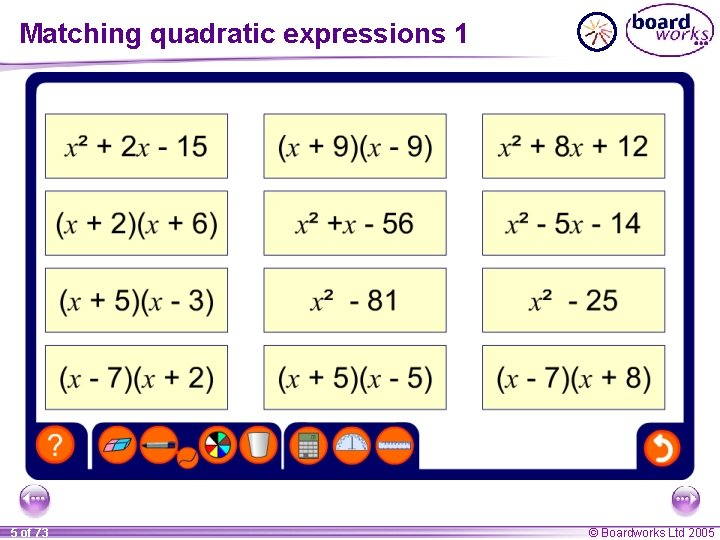Factorizing quadratic expressions Quadratic expressions of the form ax 2 + bx + c can be factorized if they can be written using brackets as (dx + e)(fx + g) where d, e, f and g are integers. If we expand (dx + e)(fx + g)we have, (dx + e)(fx + g)= dfx 2 + dgx + efx + eg = dfx 2 + (dg + ef)x + eg Comparing this to ax 2 + bx + c we can see that we must choose d, e, f and g such that: a = df, b = (dg + ef) c = eg 6 of 73 © Boardworks Ltd 2005